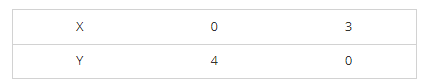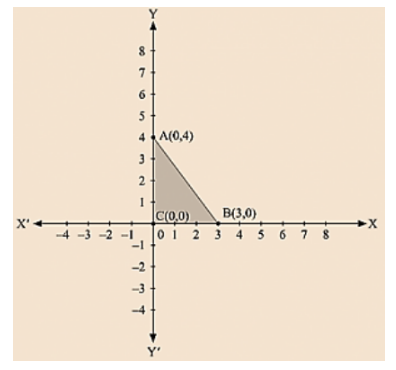# Draw the graph of the equation x/3 + y/4 = 1.

Question:

Draw the graph of the equation x/3 + y/4 = 1. Also, find the area of the triangle formed by 3/4 the line and the coordinates axes.

Solution:

We are given. x/3 + y/4 = 1

4x + 3y =12

$y=\frac{12-4 x}{3}$

Now, substituting x = 0 in

$\mathrm{y}=\frac{12-4 \mathrm{x}}{3}$

we get y = 4

Substituting x = 3 in

$y=\frac{12-4 x}{3}$

we get y = 0

Thus, we have the following table exhibiting the abscissa and ordinates of points on the line represented by the given equationThe region bounded by the graph is ABC which forms a triangle.

AC at y axis is the base of triangle having AC = 4 units on y axis.

BC at x axis is the height of triangle having BC = 3 units on x axis.

Therefore, Area of triangle ABC, say A is given by A = (Base × Height)/2

A= (AC × BC)/2

A = (4 × 3)/2

A = 6 sq. units